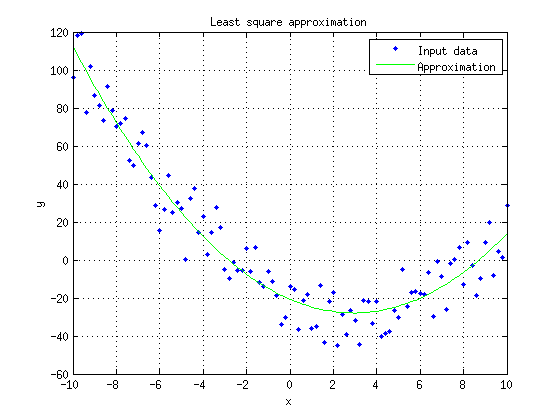Do me a favor, take a few seconds to have a look at my last project.

Thank you, Lulu

# Least square approximation with a second degree polynomial

## Hypotheses

Let's assume we want to approximate a point cloud with a second degree polynomial: $$y(x)=ax^2+bx+c$$.The point cloud is given by $$n$$ points with coordinates $${x_i,y_i}$$. The aim is to estimate $$\hat{a}$$, $$\hat{b}$$ and $$\hat{c}$$ where $$y(x)=\hat{a}x^2+\hat{b}x + \hat{c}$$ will fit the point cloud as mush as possible. We want to minimize for each point $$x_i$$ the difference between $$y_i$$ and $$y(x_i)$$, ie. we want to minimize $$\sum \limits_{i=1}^n{(y_i-y(x_i))^2}$$.

## The matrix system

The matrix form of the system is given by:

$$\left[ \begin{matrix} {x_1}^2 & x_1 & 1 \\ {x_2}^2 & x_2 & 1 \\ ... & ... & ... \\ {x_n}^2 & x_n & 1 \\ \end{matrix} \right]. \left[ \begin{matrix} \hat{a} \\ \hat{b} \\ \hat{c} \end{matrix} \right] = \left[ \begin{matrix} y_1 \\ y_2 \\ ... \\ y_n \\ \end{matrix} \right]$$

Let's define $$A$$, $$B$$ and $$\hat{x}$$:

$$\begin{matrix} A=\left[ \begin{matrix} {x_1}^2 & x_1 & 1 \\ {x_2}^2 & x_2 & 1 \\ ... & ... & ... \\ {x_n}^2 & x_n & 1 \\ \end{matrix} \right] & B=\left[ \begin{matrix} y_1 \\ y_2 \\... \\ y_n \\ \end{matrix} \right] & \hat{x}=\left[ \begin{matrix} \hat{a} \\ \hat{b} \\ \hat{c} \end{matrix} \right] \end{matrix}$$

The system is now given by:

$$A.\hat{x}=B$$

## Solving the system

The optimal solution is given by:

$$\hat{x}=A^{+}.B = A^{T}(A.A^{T})^{-1}.B$$

Where $$A^{+}$$ is the pseudoinverse of $$A$$. $$A^{+}$$ can be computed thanks to the following formula :

$$A^{+}=A^{T}(A.A^{T})^{-1}$$

## Source code

The following Matlab source code was used for drawing the above figure:

%% Matlab script for least square linear approximation
%% This script shows the approximation with a second degree polynom
%% Written by Philippe Lucidarme
%% http://www.lucidar.me
close all;
clear all;
clc;

% Set the coefficients a, b and c (y=ax²+bx+c)
a=0.8;
b=-5;
c=-20;
Noise=10;

% Create a set of points with normal distribution
X=[-10:0.2:10];
Y=a*X.*X + b*X + c + Noise*randn(1,size(X,2));

% Prepare matrices
A=[ (X.*X)' ,  X' , ones(size(X,2),1) ];
B=Y';

% Least square approximation
x=pinv(A)*B;

% Display result
plot (X,Y,'.');
hold on;
plot (X,A*x,'g');
grid on;
xlabel ('x');
ylabel ('y');
legend ('Input data','Approximation');
title ('Least square approximation');Supposons que l’on veuille approximer un nuage de points avec un polynôme du second degré : 

## Download

Matlab source code (example on this page) can be download here:

second-degree-approximation.m

## See also

Last update : 04/13/2019